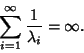## Müntz's Theorem

Müntz's theorem is a generalization of the Weierstraß Approximation Theorem, which states that any continuous function on a closed and bounded interval can be uniformly approximated by Polynomials involving constants and any Infinite Sequence of Powers whose Reciprocals diverge.

In technical language, Müntz's theorem states that the Müntz Spaceis dense inIff